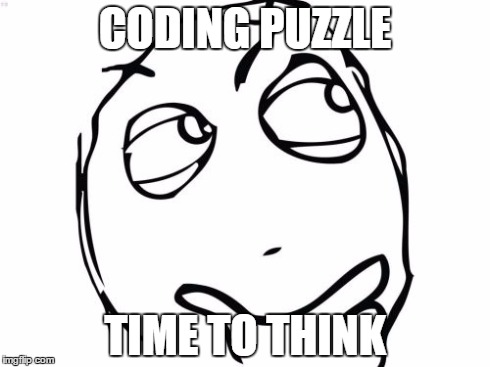# 5 Coding Puzzles Teach You How to Read Loops

#### 5 Coding Puzzles Teach You How to Read Loops

An important skill for programmers to have is to understand how code works without compiling it.

That’s why we decided to provide you with 5 coding puzzles.  Try to solve these 5 loops with your C# or other coding knowledge. Just predict the output without executing the code. If you need some help, check our C# course.  Write your answer in the comments below. Or add your own puzzle.#### 1).

int j = 0;
j = 13;
for (; j > 10; j- -)
{
Console.WriteLine(j*2);
}

#### 2).

int m = 27;
while (true)
{
Console.WriteLine(m- -);
if (m < 13)
break;
}

#### 3).

for (int k = 0; k < 10; )
{
Console.WriteLine(k++);
k++;
}

#### 4).

for (int p = 0; p < 4; p++)
{
for (int q = 0; q < 4 – p; q++)
{
Console.WriteLine(p+q);
}
}

#### 5).

int[] a = {  9, 8, 7,  10, 4, 6, 5,  1, 3, 2 };
int temp;
for (int i = 0; i < 9; i++)
{
for (int j = 0; j < 9  i; j++)
{
if (a[j] > a[j + 1])
{
temp = a[j];
a[j] = a[j + 1];
a[j + 1] = temp;
}
}
}
for (int i = 0; i < 10; i++)
{
Console.Write(a[i]);
}

Artiom Jankovskij 2015

### 1 thought on “5 Coding Puzzles Teach You How to Read Loops”

1.talkit

1. 26, 24, 22

Scroll to Top